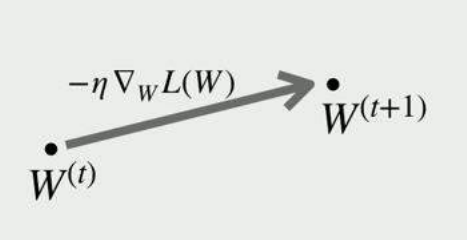# Revert optimizer.step()?

Hi everyone,

I am trying to perform the following experiment and I’d like your advice on what’s the best way to implement it in Pytorch. Given a mini-batch, weight gradients dW^{(t)} are computed based on minimizing a loss function. When we step towards that direction, we get our new weights and can calculate the new loss.What I’d like to do is based on the weight gradients (in particular, the vector direction) to step at multiple distances from W^(t), each time computing the new loss, i.e. move across the dotted line as if I am experimenting with different learning rates/step sizes and each time calculate the loss as if we were to step that much:

In other words, to call optimizer.step() but then be able to revert bach each time to the initial state, so that I can then call optimizer.step() but with a bigger step size, and to repeat this multiple times. What would the best way be to implement this?

Diagrams taken from the work of Santurkar et al. (https://papers.nips.cc/paper/7515-how-does-batch-normalization-help-optimization.pdf).

One possible approach would be to create a copy of the `state_dict` before calling the initial `step()` and then restoring the model to the initial state.
However, this should remove all `grad` attributes, so you could either store them separately or rerun the loss calculation.

@ptrblck Thanks for replying! Right now, I am doing it a bit differently (I will try your method right after). My initial learning rate was 1, so I’ve set the learning rate to 0.5, and called optimizer.step() 8 times, each time calculating the loss. However, when I try to step “back” by setting the learning rate to -3 and calling optimizer.step(), I don’t get the same loss that I had calculated in the previous step. Do you know why that would be the case?

Step 0, Accuracy 0.7734375, Loss 1.1175949573516846
Step 1, Accuracy 0.8515625, Loss 0.6774735450744629
Step 2, Accuracy 0.85546875, Loss 0.5671432614326477
Step 3, Accuracy 0.841796875, Loss 0.5469013452529907
Step 4, Accuracy 0.83203125, Loss 0.559360921382904
Step 5, Accuracy 0.80078125, Loss 0.5869375467300415
Step 6, Accuracy 0.783203125, Loss 0.6219274401664734
Step 7, Accuracy 0.76953125, Loss 0.6623443365097046

After stepping back with -3 lr: Loss: 0.7005575299263 (it should have been 0.6774735450744629)

I assumed that since I did not call loss.backwards(), the same gradients would have been applied each time. Is that incorrect?

@ptrblck When optimizer.step() is called more than once, the GPU usage increases during training until a CUDA out of memory error is thrown. Do you know why this is the case :)? Thank you!

Your approach might work, if you are using a simple optimizer, e.g. `SGD` without momentum.
When you are using momentum or an optimizer with running estimates, the reversed step might not work out of the box and you might need to look into the applied formula to check, how to revert it.

Here is a small example using `SGD`:

``````torch.manual_seed(2809)

# Set model to eval to prevent batchnorm and dropout layers of changing the output
model = models.resnet50().eval()
x = torch.randn(2, 3, 224, 224)
target = torch.randint(0, 1000, (2,))

optimizer = torch.optim.SGD(model.parameters(), lr=1e-3)
criterion = nn.CrossEntropyLoss()

# Dummy update steps
out = model(x)
loss = criterion(out, target)
loss.backward()
print('Initial loss ', loss.item())
optimizer.step()
fc0 = model.fc.weight.detach().clone()

# Get updated loss
out = model(x)
loss = criterion(out, target)
print('Updated loss ', loss.item())
fc1 = model.fc.weight.detach().clone()

# Use negative lr
optimizer.param_groups['lr'] = -1. * optimizer.param_groups['lr']
optimizer.step()
out = model(x)
loss = criterion(out, target)
print('Reverted loss ', loss.item())
fc2 = model.fc.weight.detach().clone()
``````

`optimizer.step()` should not increase the memory usage. Are you sure this line causes the OOM issue?
Usually you would run out of memory, if you are storing some tensors, which are not detached from the computation graph.

4 Likes

If I don’t call loss.backwards after optimizer.step(), the GPU usage keeps on increasing.

``````for step_index in range(8):
optimizer.step()
outputs = model(X)
loss = loss_function(outputs, y)
loss.backward() // If I comment out, GPU usage increases until OOM error

if step_index == 1:
'model': model.state_dict()
}

``````

Did u forget to call `optimizer.zero_grad()`? I think that if u don’t call it the gradients will just stack up and increase the memory usage.

Are you storing the loss or gradients somehow?
The gradients should be accumulated, so the memory footprint should not increase.

2 Likes

You were right. I was storing the gradients. Thank you! It works nowNote that I used the negative learning rate approach.

Now, the next step is to compute new gradients at each point along the gradient vector line. However, loss.backwards is not an option as that would update the model. Is there any way of computing the current gradients using a function that returns them, rather than changing them in-place?

You’ll need need to hack it by updating parameters for each evaluation point and then restoring to original values. It’s kind of inherent to structure of torch.nn – since parameters are a state you need to modify state. Parameter=state structure makes it easier for a common cases like SGD, but awkward when you need to try several parameter values per step (ie, line-search or bayesian optimization)

1 Like

What if I clone the model and do loss.backwards on that model?

``````for step_index in range(8):
optimizer.step()
temp_model = copy.deepcopy(model)
temp_outputs = temp_model(X)
cur_loss = loss_function(temp_outputs, y)
temp_loss = cur_loss.item()
cur_loss.backward()
``````

Would that be an overkill? Also, since the goal is to calculate the L2 norm between these new gradients and the initial gradients, I could then just do:

``````    temp_totall2norm = 0
for i, p1 in enumerate(model.parameters()):
for j, p2 in enumerate(temp_model.parameters()):
if i != j:
continue